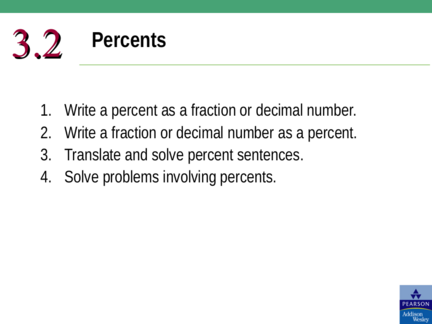# Percentage and its Conversion into different FormsContributed by:This pdf includes the following topics:-
Percentage
Percents to Decimals
Percents to Fractions
Decimals to Percents
Fractions to Percents
Percent Sentences
1. 3.2 Percents
1. Write a percent as a fraction or decimal number.
2. Write a fraction or decimal number as a percent.
3. Translate and solve percent sentences.
4. Solve problems involving percents.
2. Ratio: A comparison of two quantities using a quotient (fraction).
a c
Proportion: Two ratios set equal. 
b d
Cross Cross products are equal. ad  bc
Percent: A ratio representing some part out of 100.
20% = 20 out of 100
20

100
1
 or 0.2
5
3. Percents to Decimals
Drop % and move decimal point 2 places to the left.
55% =.55
38% =.38
14.5% =.145
.25% =.0025
1
45 %  45.5%  .455
2
4. Percents to Fractions
Change to a decimal, then Read It, Write It, Reduce It
55 11
55% =.55 
100

20
38 19
38% =.38  
100 50
145 29
14.5% =.145  
1000 200
25 1
.25% =.0025  10000 
400
1 455 91
45 %  45.5%  .455  
2 1000 200
5. Decimals to Percents
Move the decimal point 2 places to the right and add %.
.55 = 55%
.38 = 38%
2.5 = 250%
.003 = .3%
6. Fractions to Percents
Change to a decimal and then to a percent.
3
 .75 75%
4
5
 .625 62.5%
8
2
3 3.4 340%
5
7. What is 7/8 as a percent?
a) 78%
b) 87%
c) 87.5%
d) 718%
8. What is 7/8 as a percent?
a) 78%
b) 87%
c) 87.5%
d) 718%
9. Percent Sentences
A percent of a whole is a part of the whole.
% multiply total = part
(follows “of”)
36% of 72 is what number?
.36 ∙ 72 = x
.36 (72) = x
25.92 = x
36% of 72 is 25.92.
10. Percent Sentences
A percent of a whole is a part of the whole.
% multiply total = part
42% of what number is 31.92?
.42 ∙ x = 31.92
.42 x = 31.92
x = 76
42% of 76 is 31.92.
11. Percent Sentences
What percent of 63 is 56?
x ∙ 63 = 56
63 x = 56
x = .8888888…
x = 88.9%
88.9% of 63 is 56.
12. 2.4% of 130 is what number?
a) 31.2
b) 3.12
c) 1.83
d) 0.18
13. 2.4% of 130 is what number?
a) 31.2
b) 3.12
c) 1.83
d) 0.18
14. What percent of 48 is 51.84?
a) 93%
b) 98%
c) 108%
d) 109%
15. What percent of 48 is 51.84?
a) 93%
b) 98%
c) 108%
d) 109%
16. Percent Sentences
On Monday, January 17, 2012, the Morning Sentinel
reported it planned to cut 100 full-time jobs in a bid
newspaper’s workforce. How many employees does
the Sentinel have?
Percent: 6% = .06 Percent ∙ whole = part
Whole: x .06 x = 100
Part: 100 x = 1666.66666
x = 1667
Sentinel has 1,667 employees.
17. Percent Sentences
Jamal purchases a new surfboard that costs \$570.
The sales tax is 7%, what is the total cost of the
surfboard?
Percent: 7% = .07 Percent ∙ whole = part
Whole: 570 .07(570) = x
Part: x x = 39.90
What did we find? Sales tax. 570.00
39.90
Total cost = cost plus tax. 609.90
Total cost is \$609.90.